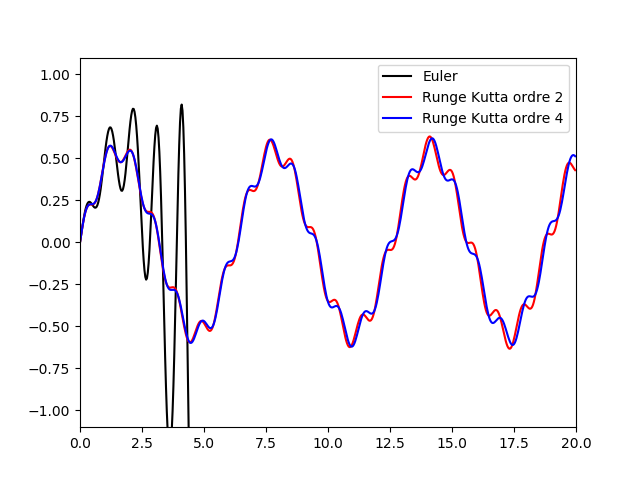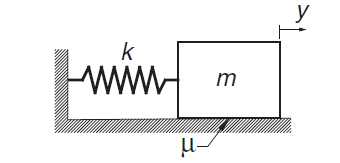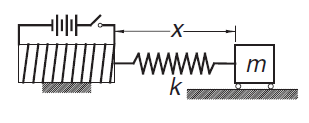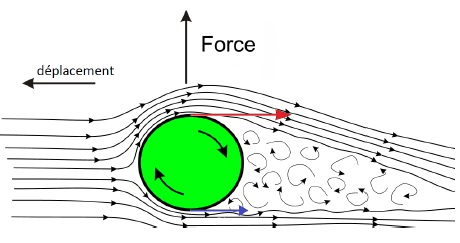$$\newcommand{\dint}{\mathrm{d}} \newcommand{\vphi}{\boldsymbol{\phi}} \newcommand{\vpi}{\boldsymbol{\pi}} \newcommand{\vpsi}{\boldsymbol{\psi}} \newcommand{\vomg}{\boldsymbol{\omega}} \newcommand{\vsigma}{\boldsymbol{\sigma}} \newcommand{\vzeta}{\boldsymbol{\zeta}} \renewcommand{\vx}{\mathbf{x}} \renewcommand{\vy}{\mathbf{y}} \renewcommand{\vz}{\mathbf{z}} \renewcommand{\vh}{\mathbf{h}} \renewcommand{\b}{\mathbf} \renewcommand{\vec}{\mathrm{vec}} \newcommand{\vecemph}{\mathrm{vec}} \newcommand{\mvn}{\mathcal{MN}} \newcommand{\G}{\mathcal{G}} \newcommand{\M}{\mathcal{M}} \newcommand{\N}{\mathcal{N}} \newcommand{\S}{\mathcal{S}} \newcommand{\diag}{\mathrm{diag}(#1)} \newcommand{\diagemph}{\mathrm{diag}(#1)} \newcommand{\tr}{\text{tr}(#1)} \renewcommand{\C}{\mathbb{C}} \renewcommand{\R}{\mathbb{R}} \renewcommand{\E}{\mathbb{E}} \newcommand{\D}{\mathcal{D}} \newcommand{\inner}{\langle #1 \rangle} \newcommand{\innerbig}{\left \langle #1 \right \rangle} \newcommand{\abs}{\lvert #1 \rvert} \newcommand{\norm}{\lVert #1 \rVert} \newcommand{\two}{\mathrm{II}} \newcommand{\GL}{\mathrm{GL}} \newcommand{\Id}{\mathrm{Id}} \newcommand{\grad}{\mathrm{grad} \, #1} \newcommand{\gradat}{\mathrm{grad} \, #1 \, \vert_{#2}} \newcommand{\Hess}{\mathrm{Hess} \, #1} \newcommand{\T}{\text{T}} \newcommand{\dim}{\mathrm{dim} \, #1} \newcommand{\partder}{\frac{\partial #1}{\partial #2}} \newcommand{\rank}{\mathrm{rank} \, #1}$$

# Solving Ordinary Non Linear Differential Equations

## Introduction

Let $\left[ a,b\right]$ be in $\mathbb{R}$ and $y_{0}$ be a real value. Then we define function $f$: $% \begin{array} [c]{c}% f:\left[ a,b\right] \times\mathbb{R}\rightarrow\mathbb{R}\\ \left( t,y\right) \mapsto f\left( t,y\right) \end{array}$ We wish to solve the following Cauchy problem, for all: \begin{align*} & \left\{ \begin{array} [c]{l}% y^{\prime}\left( t\right) =f\left( t,y\right) \;t \in\left[ a,b\right] \\ y\left( a\right) =y_{0}% \end{array} \right. \\ \end{align*} We have to find a continuous function $y$, derivable on $\left[ a,b\right]$ such that it yields: $for\;all\; t\in\left[ a,b\right],\;y^{\prime}\left( t\right) =f\left( t,y\left( t\right) \right)$ with $y\left( a\right) =y_{0}.$

Function $y\left( t\right) =\dfrac{t^{2}}{4}$ is a solution of the following problem: $$\left\{ \begin{array} [c]{l}% y^{\prime}\left( t\right) =\sqrt{y(t)}\text{ pour }t\in\left[ 0,4\right] \\ y\left( 0\right) =0 \end{array} \right.$$ Nevertheless, we can show that for any $\alpha>0$ functions: $\left\{ \begin{array} [c]{l}% y\left( t\right) =0\text{ for }t\in\left[ 0,\alpha\right] \\ y\left( t\right) =\dfrac{\left( t-\alpha\right) ^{2}}{4}\text{ si }t>\alpha \end{array} \right.$ are also solutions to this Cauchy problem... The solution is not unique.

Function $f:\left[ a,b\right] \times\mathbb{R}\rightarrow\mathbb{R}$ is said lipschitz in $y$ if there exists a real value $L>0$ called Lipschitz's constant such that for all $t\in\left[ a,b\right]$, $y_{1}$ and $y_{2}$ of $\mathbb{R}$ we have $\left\| f\left( t,y_{1}\right) -f\left( t,y_{2}\right) \right\| \leq L.\left\| y_{1}-y_{2}\right\|$
[Cauchy-Lipschitz]. Let $\left( \mathcal{C}\right) \;\left\{ \begin{array} [c]{l}% y^{\prime}\left( t\right) =f\left( t,y\right) \\ y\left( a\right) =y_{0}% \end{array} \right.$ be a Cauchy problem. If $f:\left[ a,b\right] \times\mathbb{R}\rightarrow\mathbb{R}$ satisfies the following assumptions
• $f$ is continuous on $\left[ a,b\right]\times\mathbb{R}$
• $f$ is lipschitz on $y$
then the Cauchy problem has a unique solution of class C$^{1}$.

## Euler Algorithm (1768)

In general, we can't find the exact solution $y$ of the Cauchy problem $\mathcal{C}$. It's why we try to find an approximation $y_{1}$ of $y\left( t_{0}+h\right) =y\left( t_{1}\right)$ where $h>0$ is the step of the numerical method. We set $t_{i}=t_{i-1}+h$ for $i=1,\ldots,n$ with $t_{0}=a$ and $t_{n}=b.$ The Euler algorithm try to approximate the exact solution of $y\left( t_{0}+h\right)$ at $t_{0}+h$ by using the tangent value of $y\left(t\right)$ at $\left( t_{0},y_{0}\right) .$ The tangent equation can be written by: $y-y_{0}=y^{\prime}\left( t_{0}\right) \left( x-t_{0}\right)$ Hence, for $x=t_{0}+h$ we have: $y_{1}=y_{0}+f\left( t_{0},y_{0}\right) .h$ We then solve this new Cauchy problem: $\left( \mathcal{C}_{1}\right) \;\left\{ \begin{array} [c]{l}% y^{\prime}\left( t\right) =f\left( t,y\right) \\ y\left( t_{1}\right) =y_{1}% \end{array} \right.$ which allows us to approximate $y(t_2)$ by $y_{2}$ at $y\left( t_{1}+h\right) :$ $y_{2}=y_{1}+h.f\left( t_{1},y_{1}\right).$ Iteratively, we obtain the $n$ values $y_{i}$ which approximate function $y$ at each $t_{i}$.

Exercise [Non Linear Equation of order $1$]
Let us given the following Cauchy problem: \begin{equation} \quad \left\{ \begin{array}{lll} y'(t) & = & 1 + (y(t))^2, \quad t\in [0,1],\\ y(0) & = & 0.\\ \end{array}\right. \end{equation}

• Solve this Cauchy problem with the scalar Euler algorithm. Draw the solution curve with Python. For this you have to define the following function:

• 
import numpy as np
import matplotlib.pyplot as plt

""" ODE function """
def f(t,y):
return 1+y*y

""" Euler Algorithm"""
def Euler(y0,a,b,nb):
h=(b-a)/nb
t=a
X=[a]
Y=[y0]
y1=y0
for i in range(1,nb):
#TO DO
Y.append(y2)
X.append(t)
y1=y2
return X,Y

y0= 0.0
a = 0.0
b = 1.0
niter = 150
x,y = Euler(y0,a,b,niter)
plt.plot(x,y,'r', label=r"Euler method", linestyle='--')
plt.show()


• Solve this Cauchy problem with the scalar Runge-Kutta of order $2$ algorithm (see Runge Kutta Algorithms ).

• Solve this Cauchy problem with the scalar Runge-Kutta of order $4$ algorithm.

• Solve this Cauchy problem with the second order Adams-Moulton algorithm.

Exercise [Ricatti (1712) Non Linear Equation of order $1$]
Let us given the following Cauchy problem: \begin{equation} \quad \left\{ \begin{array}{lll} y'(t) & = & t^2 + (y(t))^2, \quad t\in \left[0,\dfrac{1}{2}\right],\\ y(0) & = & 0.\\ \end{array}\right. \end{equation}

• Solve this Cauchy problem with the scalar Euler and Runge-Kutta algorithm.

• Draw the solution curves with Python.

• Compare the approximted values of $y_{nb}$ with the reference solution
$y(\frac{1}{2}) =$ 0.041 791 146 154 681 863 220 76

Exercise [Numerical instability]
Let us given the following Cauchy problem: \begin{equation} \quad \left\{ \begin{array}{lll} y'(t) & = & 3y(t) - 4e^{-t}, \quad t\in [0,10],\\ y(0) & = & 1.\\ \end{array}\right. \end{equation}

1. Use the fourth-order Runge-Kutta method with step $h=0.1$ to solve this problem.
2. Compare the result with the analytical solution $y(t) = e^{-t}$.

Solving a second order differential Cauchy problem

Let us give $(D)\left\{ \begin{array} [c]{l}% y^{\prime\prime}=f\left( t,y,y^{\prime}\right) \\ y\left( t_{0}\right) =y_{0}\\ y^{\prime}\left( t_{0}\right) =y_{0}^{\prime}% \end{array} \right.$ where function $f$ is of class $C^{1}$. By setting $V\left( t\right) =\left( \begin{array} [c]{c}% y\left( t\right) \\ y^{\prime}\left( t\right) \end{array} \right)$ we obtain the following first order Cauchy problem: $\left\{ \begin{array} [c]{l}% \dfrac{dV\left( t\right) }{dt}=\left( \begin{array} [c]{c}% y^{\prime}\\ y^{\prime\prime}% \end{array} \right) =\left( \begin{array} [c]{c}% y^{\prime}\\ f\left( t,y,y^{\prime}\right) \end{array} \right) =F\left( t,V\right) \\ V\left( t_{0}\right) =\left( \begin{array} [c]{c}% y_{0}\\ y_{0}^{\prime}% \end{array} \right) \end{array} \right.$ Thus, we can solve this problem by using the Euler algorithm where the first iteration is given by: $V_{1}=V_{0}+h.F\left( t_{0},V_{0}\right)$

Exercise [Non Linear Equation of order $2$]
We want to solve this second order non linear differential equation: $\left \{ \begin{array}{l} ml^{2}\theta ^{\prime \prime }\left( t\right) =-mgl\sin \left( \theta \left( t\right) \right) +c\left( t\right) \text{ pour }t\in \left[ 0,T\right] , \\ \theta \left( 0\right) =0 \\ \theta ^{\prime }\left( 0\right) =1.% \end{array}% \right.$ with torque $c\left( t\right) =A\sin \left( t\right)$, $m=0.1$kg, $l=30$cm, $T=5$s and $A=0.1$N.m.

1. Solve this Cauchy problem with the Euler vector algorithm.

2. 
import numpy as np

""" Euler Vector Algorithm"""

def F(t,V):
L=[V, # TO DO]
return np.array(L)

def NEuler(y0,a,b,nb):
h=(b-a)/nb
t=a
X=[a]
Y=[V0]
V1=V0
for i in range(1,nb):
#TO DO
t=t+h
Y.append(V2)
X.append(t)
V1=V2
return X,Y


3. Solve this Cauchy problem with the Runge-Kutta of order $2$ algorithm.

4. Solve this Cauchy problem with the Runge-Kutta of order $4$ algorithm.

5. Solve this Cauchy problem with the second order Adams-Moulton algorithm.Exercise [Mass Spring]Consider the mass–spring system where dry friction is present between the block and the horizontal surface. The frictional force has a constant magnitude $\mu.m.g$ ($\mu$ is the coefficient of friction) and always opposes the motion. We denote by $y$ the measure from the position where the spring is unstretched.

We assume that $k = 3000$N/m, $\mu = 0.5$, $g = 9.80665$m/s², $y_0=0.1$m and $m= 6.0$ kg.
1. Write the differential equation of the motion of the block
2. If the block is released from rest at $y = y_0$, verify by numerical integration that the next positive peak value of $y$ is $y_0 − 4\mu.m.\frac{g}{k}$. This relationship can be derived analytically.

Exercise [Iron block]The magnetized iron block of mass $m$ is attached to a spring of stiffness $k$ and free length $L$. The block is at rest at $x = L$. When the electromagnet is turned on, it exerts a repulsive force $F = c/x^2$ on the block.

We assume that $c = 5$N·m², $k = 120$ N/m, $L = 0.2$m and $m= 1.0$ kg.
1. Write the differential equation of the motion of the mass
2. Determine the amplitude and the period of the motion by numerical integration

Exercise [Magnus Effect applied to a ball]
On June 3, 1997, Roberto Carlos scored against France football team a free kick goal with an improbable effect. We want to simulate in this exercice what happened that day. For this, we set:

• $\rho=1.2 \ Kg.m^{-3}$ : the air density
• $R=0.11\ m$ the radius of the ball
• $m = 0.430\ Kg$ : the mass of the ball
• $M(t)=(x(t),y(t),z(t))$: the location of the ball at $t\ge 0$
• $v_0=36 \ m.s^{-1}$ : the initial speed of the ball
• $v(t)$ : the speed of the ball at $t\ge 0$.
• $\overrightarrow{v}(t)$ : the speed vector of the ball at $t\ge 0$.
• $\overrightarrow{\omega}=(0,0,\omega_0)$ : the angular velocity of the ball which is assumed to be constant during the trajectory
• $\alpha$ : the angle between $\overrightarrow{\omega}$ and $\overrightarrow{v}$
• $\beta$, $\gamma$ : are the initial angles of the force applied to the ballWe assume that the forces applied to the ball are
• its weight
• a friction force: $\overrightarrow{f}$, $\Vert \overrightarrow{f} \Vert=\frac{1}{2}C_f\pi R^2\rho v^2,$ where $C_f\approx 0.45$
• a lift force $\overrightarrow{F}=\frac{1}{2}\pi\rho R^3\sin(\alpha)\ \overrightarrow{\omega}\wedge \overrightarrow{v}$.
1. Define the differential equation apply to the ball.

2. Define the initial conditions.

3. Solve this Cauchy problem with the Runge-Kutta of order $4$ algorithm.

4. Solve this Cauchy problem with the second order Adams-Moulton algorithm.

## Runge-Kutta Algorithms

Runge-Kutta of order $2$

We want to solve the following Cauchy problem: $\left\{ \begin{array} [c]{l}% y^{\prime}\left( t\right) =f\left( t,y\right) \\ y\left( t_{0}\right) =y_{0}% \end{array} \right. \text{ for }t\in\left[ t_{0},t_{n}\right]$ where $f$ is of class $C^{1}$. For this, we set \begin{align*} k_{1} & =h.f\left( t_{0},y_{0}\right) \\ k_{2} & =h.f\left( t_{0}+ah,y_{0}+\alpha k_{1}\right) \end{align*} and $y_{1}=y_{0}+R_{1}k_{1}+R_{2}k_{2}%$ Consequently, we have to calculate the unknows $a,\alpha,R_{1},R_{2}$ such that we minimize the following error: $e_{1}=y\left( t_{1}\right) -y_{1}%$ To do so, we identify the Taylor expansion of $y\left( t_{1}\right)$ and $y_{1}.$ We assume that $f$ is of class $C^{2}$. As \begin{align*} \frac{dy}{dt} & =f\left( t,y\right) \\ \frac{d^{2}y}{dt^{2}} & =\frac{\partial f\left( t,y\right) }{\partial t}+\frac{\partial f\left( t,y\right) }{\partial y}f\left( t,y\right) \end{align*} it yields, \begin{align*} y\left( t_{1}\right) & =y\left( t_{0}+h\right) \\ & \\ y\left( t_{1}\right) & =y_{0}+h.\frac{dy\left( t_{0}\right) }{dt}% +\frac{h^{2}}{2}\frac{d^{2}y\left( t_{0}\right) }{dt^{2}}+O\left( h^{3}\right) \\ & \\ y\left( t_{1}\right) & =y_{0}+h.f\left( t_{0},y_{0}\right) +\frac{h^{2}% }{2}\left[ \frac{\partial f\left( t_{0},y_{0}\right) }{\partial t}% +\frac{\partial f\left( t_{0},y_{0}\right) }{\partial y}f\left( t_{0}% ,y_{0}\right) \right] +O\left( h^{3}\right) \end{align*} As $\varphi\left(t,y,h\right) =R_{1}k_{1}+R_{2}k_{2}$ is of class $C^{2}$, we can apply the Taylor expansion at $h=0$. Then we have, \begin{align*} \varphi\left( t,y,h\right) & =\varphi\left( t,y,0\right) +h\frac {\partial\varphi\left( t,y,0\right) }{\partial h}+\frac{h^{2}}{2}% \frac{\partial^{2}\varphi\left( t,y,0\right) }{\partial h^{2}}+O\left( h^{3}\right) \\ \varphi\left( t,y,h\right) & =0+h\left[ R_{1}.f\left( t_{0}% ,y_{0}\right) \right] +h.\left[ R_{2}.f\left( t_{0},y_{0}\right) +R_{2}ha\frac{\partial f\left( t_{0},y_{0}\right) }{\partial t}\right] \\ & +h\left[ R_{2}h\alpha\frac{\partial f\left( t_{0},y_{0}\right) }{\partial y}f\left( t_{0},y_{0}\right) \right] +O\left( h^{3}\right) \end{align*} It comes $y_{1}=y_{0}+\left( R_{1}+R_{2}\right) hf\left( t_{0},y_{0}\right) \\ +h^{2}\left[ aR_{2}\frac{\partial f\left( t_{0},y_{0}\right) }{\partial t}+\alpha R_{2}\frac{\partial f\left( t_{0},y_{0}\right) }{\partial y}f\left( t_{0},y_{0}\right) \right] +O\left( h^{3}\right) .$ Then, the difference \begin{align*} y\left( t_{1}\right) -y_{1} & =\left( 1-R_{1}-R_{2}\right) hf\left( t_{0},y_{0}\right) +h^{2}\left[ \left( \frac{1}{2}-aR_{2}\right) \frac{\partial f\left( t_{0},y_{0}\right) }{\partial t}\right] \\ & +h^{2}\left[ \left( \frac{1}{2}-\alpha R_{2}\right) \frac{\partial f\left( t_{0},y_{0}\right) }{\partial y}f\left( t_{0},y_{0}\right) \right] +O\left( h^{3}\right) \end{align*} is of order $O\left( h^{3}\right)$ if $R_{1}+R_{2}=1$ and $aR_{2}=\alpha R_{2}=\dfrac{1}{2}.$ It follows that:

• if $R_{2}=0$ then we obtain the previous Euler method
• if $R_{1}=0,R_{2}=1$ and $a=\alpha=\dfrac{1}{2}$ we obtain the modified Euler method where $y_{1}=y_{0}+h.f\left( t_{0} +\frac{h}{2},y_{0}+\frac{h}{2}f\left( t_{0},y_{0}\right) \right)$
• if $R_{1}=R_{2}=\dfrac{1}{2}$ and $a=\alpha=1$ then we obtain the Euler-Cauchy method where $y_{1}=y_{0}+\dfrac{1}{2}hf\left( t_{0},y_{0}\right) +\dfrac{1}{2}h.f\left( t_{0}+h,y_{0}+hf\left( t_{0},y_{0}\right) \right)$.

Runge-Kutta of order $4$

The fourth-order Runge–Kutta method is obtained from the Taylor series along the samelines as the second-ordermethod. Since the derivation is rather long and not very instructive, we skip it. The final form of the integration formula again depends on the choice of the parameters; that is, there is no unique Runge–Kutta fourth-order formula. The most popular version, which is known simply as the Runge–Kutta method, entails the following sequence of operations: \begin{align*} k_{1} & =h.f\left( t_{0},y_{0}\right) \\ k_{2} & =h.f\left( t_{0}+\frac{h}{2},y_{0}+\frac{k_{1}}{2}\right) \\ k_{3} & =h.f\left( t_{0}+\frac{h}{2},y_{0}+\frac{k_{2}}{2}\right) \\ k_{4} & =h.f\left( t_{0}+h,y_{0}+k_{3}\right) \\ y_{1} & =y_{0}+\frac{1}{6}\left( k_{1}+2k_{2}+2k_{3}+k_{4}\right) \end{align*} The main drawback of this method is that it does not lend itself to an estimate of the truncation error. Therefore, we must guess the integration step size $h$, or determine it by trial and error. In contrast, the so-called adaptive methods can evaluate the truncation error in each integration step and adjust the value of $h$ accordingly (but at a higher cost of computation). The adaptive method is introduced in the next Section.

## Multi-step Algorithms

For all $n$, we set $f\left( t_{n},y_{n}\right) =f_{n}.$ We call $k$-step method an algorithm of the kind: $\left\{ \begin{array} [c]{c}% y_{n}=\sum_{i=1}^{k}\alpha_{i}y_{n-i}+h \sum^{k}_{i=0}\beta_{i}f_{n-i}\text{ for }n\geq k\\ y_{0,}\ldots y_{k-1}\text{ are given} \end{array} \right.$ where the $\alpha_{i}$ and $\beta_{i}$ are real values which are not dependant of $h$.

• If $\beta_{0}=0$ then we have an explicit method.
• If $\beta_{0}\neq0$ then we have an implicit method.
For example, $y_{n}=\frac{4}{3}y_{n-1}-\frac{1}{3}y_{n-2}+\frac{2h}{3}f_{n}%$ is implicit and needs $y_{0}$ and $y_{1}$. The value $y_{n}$ is given by using a fixed-point methodology or by a prediction - correction methodology.

If we integrate the differential equation we have: $y\left( t_{n}\right) =y\left( t_{n-1}\right) +\int_{t_{n-1}}^{t_{n}% }f\left( t,y\left( t\right) \right) dt$ In order to calculte this integral, we substitute $f\left( t,y\left( t\right) \right)$ by an interpolation polynomial $p\left( t\right)$ of degree $k$ which satisfies $p\left( t_{j}\right) =f\left( t_{j}% ,y_{j}\right) =f_{j}$ for $j=n-k,\ldots,n$. For this, we need to take into account the $k$ values $y_{n-k},\ldots,y_{n-1}$ that were calculated previously and $y_{n}$ which is known. If we write this polynomial in the Newton'polynomial basis we obtain: $y_{n}=y_{n-1}+\sum_{j=0}^{k}\left( \prod_{i=0}^{j-1}\left( t-t_{n-i}\right) f\left[ t_{n},\ldots,t_{n-j}\right] \right) .$ If the abscissa $t_{j}$ are uniform then it yields: $y_{n}=y_{n-1}+h\underset{i=0}{\overset{k}{\sum}}\beta_{i}f_{n-i}\text{.}%$ Coefficients $\beta_{i}$ have to be defined such that the formula are exacts for maximum degree polynomials. If $k=2$, \begin{align*} \text{for }y(t) & =1\text{ then }y^{\prime}(t)=0\text{ }\\ \text{and }y\left( t_{n}\right) & =1=y\left( t_{n-1}\right) +h.(f\left( t_{n},y_{n}\right) .\beta_{0}+f\left( t_{n-1},y_{n-1}\right) .\beta _{1}+f\left( t_{n-2},y_{n-2}\right) .\beta_{2})\\ \text{It yields }1 & =1+h.\left[ 0.\beta_{0}+0.\beta_{1}+0.\beta_{2}\right] \end{align*} \begin{align*} \text{for }y(t) & =t-t_{n}\text{ then }y^{\prime}(t)=1\\ \text{and }y\left( t_{n}\right) & =0=-h+h.(\beta_{0}+\beta_{1}+\beta_{2}) \end{align*} \begin{align*} \text{for }y(t) & =\left( t-t_{n}\right) ^{2}\text{ then }y^{\prime }(t)=2\left( t-t_{n}\right) \\ \text{and }y\left( t_{n}\right) & =0=h^{2}+h.(0.\beta_{0}-2h.\beta _{1}-4h.\beta_{2}) \end{align*} From \begin{align*} \beta_{0}+\beta_{1}+\beta_{2} & =1\\ 2.\beta_{1}-4.\beta_{2} & =1 \end{align*} we obtain that \begin{align*} \beta_{0} & =\beta_{1}=\frac{1}{2}\\ \beta_{2} & =0 \end{align*} Consequently, the Adams-Moulton of order $2$ can be written: $y_{n}=y_{n-1}+\frac{h}{2}\left( f_{n}+f_{n-1}\right)$ Order 3: $y_{n}=y_{n-1}+\frac{h}{12}\left( 5f_{n}+8f_{n-1}-f_{n-2}\right)$
• To calculate $f_{n}$ we need to know the value $y_{n}$ then we have implicit methods. To solve then, we can approximate $y_{n}$ by $y_{n}^{\ast}$ by using an explicit method and insert this value in the implicit schema (correction step).
Order 2 : $y_{n}=y_{n-1}+\frac{h}{2}\left( 3f_{n-1}-f_{n-2}\right)$ Order 3 : $y_{n}=y_{n-1}+\frac{h}{12}\left( 23f_{n-1}-16f_{n-2}+5f_{n-3}\right)$